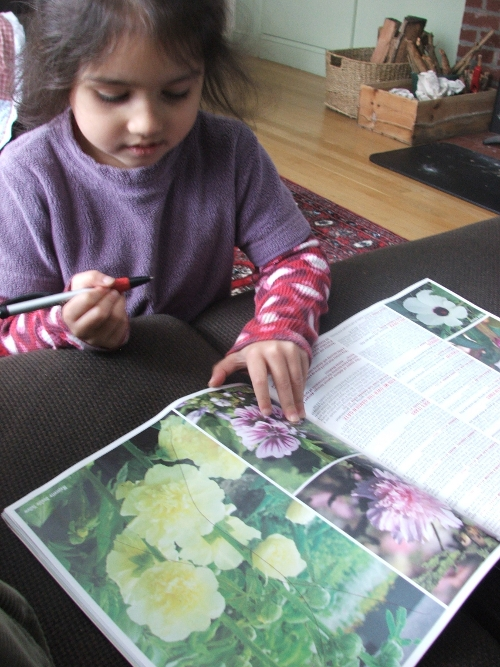# Oh My.Amie chooses flowers

I put in my seed order. I may have gone overboard a bit, again. No, honestly now, not “again”: even more so, than last year. And these are seeds over and above what I still have left. To be honest I am ashamed to publish the list. Oh my.

But, truth be told, there will be much more space this time around. Last year we missed out on the entire Spring season in the vegetable garden. We also didn’t have those large beds up front, where I can finally put the herbs and the perennials. Planning and planting those will be a whole new ballgame for me. Oh my.

I haven’t looked at flowers yet. Or berry bushes. Or nut and fruit trees. Oh my.

~

Okay. Here goes, my Fedco order:

205PO-Provider Bush Green Bean OG (A=2oz) 1 x \$1.80= \$1.80
249MO-Maxibel Bush Haricots Verts OG (A=1/2oz) 1 x \$1.00= \$1.00
299WI-Windsor Fava Bean (A=2oz) 1 x \$1.40= \$1.40
323JW-Jackson Wonder Bush Lima Bean (A=2oz) 1 x \$1.20= \$1.20-currently on backorder
332CN-Cannellini Bean (A=2oz) 1 x \$1.50= \$1.50
336KE-King of the Early Bean (A=2oz) 1 x \$1.40= \$1.40
343JC-Jacobs Cattle Bean (A=2oz) 1 x \$1.90= \$1.90
376RK-Red Kidney Bean (A=2oz) 1 x \$1.40= \$1.40
732FR-Early Frosty Shell Pea (A=2oz) 1 x \$1.20= \$1.20
748LM-Little Marvel Shell Pea (A=2oz) 1 x \$1.20= \$1.20
761GO-Green Arrow Shell Pea OG (A=2oz) 1 x \$1.80= \$1.80
798LG-Legume Inoculant (A=treats 8lb) 1 x \$4.00= \$4.00
822BO-Blizzard Snow Pea OG (A=2oz) 1 x \$2.00= \$2.00
893SO-Sugarsnap Snap Pea OG (A=2oz) 1 x \$2.00= \$2.00
1382SZ-Super Zagross Middle Eastern Slicing Cucumber (A=1/16oz) 1 x \$1.30= \$1.30
1688WO-Waltham Butternut Winter Squash OG (A=1/4oz) 1 x \$1.20= \$1.20-currently on backorder
1705JL-Jack Be Little Pumpkin (A=1/8oz) 1 x \$1.30= \$1.30
2018TP-Tonda di Parigi Carrot (A=1/8oz) 1 x \$1.20= \$1.20-currently on backorder
2300TG-Takinogawa Burdock (A=1/8oz) 1 x \$1.20= \$1.20
2407RO-King Richard Leek OG (A=1/16oz) 1 x \$1.60= \$1.60
2439EV-Evergreen Hardy White Scallion (A=1/16oz) 1 x \$1.20= \$1.20
2444CW-Crystal White Wax Onion (A=1/16oz) 1 x \$1.20= \$1.20
2474DO-Clear Dawn Onion OG (A=1/16oz) 1 x \$1.40= \$1.40
2510SP-Space Spinach (A=1/4oz) 1 x \$1.00= \$1.00
2555GW-Giant Winter Spinach (A=1/4oz) 1 x \$1.10= \$1.10-currently on backorder
2803TT-Tom Thumb Lettuce (A=2g) 1 x \$0.70= \$0.70
2865RH-Rouge dHiver Lettuce (A=2g) 1 x \$0.70= \$0.70
2986SM-Summer Lettuce Mix (A=1g) 1 x \$1.10= \$1.10
3036BL-Bright Lights Chard (A=1/16oz) 1 x \$1.10= \$1.10
3048PZ-Pan di Zucchero Chicory (A=0.5g) 1 x \$1.20= \$1.20
3058WC-Watercress (A=1/16oz) 1 x \$1.30= \$1.30-currently on backorder
3096KH-Good King Henry Chenopodium or Goosefoot (A=0.5g) 1 x \$1.30= \$1.30
3099SK-Sea Kale (A=1g) 1 x \$2.00= \$2.00
3122MN-Minutina (A=1/16oz) 1 x \$1.30= \$1.30
3168KR-Krausa Parsley (A=1/16oz) 1 x \$1.00= \$1.00
3192BL-Broad-Leaved Sorrel (A=1/16oz) 1 x \$0.90= \$0.90
3320WM-Waltham 29 Broccoli (A=2g) 1 x \$0.70= \$0.70
3336RN-Roodnerf Brussels Sprouts (A=2g) 1 x \$1.10= \$1.10
3404CS-Charming Snow Cauliflower (A=0.5g) 1 x \$1.20= \$1.20
3445CO-EvenStar Champion Collards OG (A=2g) 1 x \$2.00= \$2.00
3463WB-Winterbor Kale (A=0.5g) 1 x \$1.80= \$1.80
3616SF-Safir Cutting Celery (A=0.2g) 1 x \$1.20= \$1.20
3644DC-Diamante Celeriac (A=0.2g) 1 x \$2.40= \$2.40-replaced with Brilliant
3684DM-Diamond Eggplant (A=0.2g) 1 x \$0.90= \$0.90
3704PO-Peacework Sweet Pepper OG (A=0.2g) 1 x \$1.00= \$1.00
3753BO-Czech Black Hot Pepper OG (A=0.2g) 1 x \$1.60= \$1.60
3810AC-New Ace Sweet Pepper (A=0.2g) 1 x \$1.60= \$1.60
4008HC-Husk Cherry (A=0.5g) 1 x \$1.00= \$1.00
4059CO-Cherokee Purple Tomato OG (A=0.2g) 1 x \$1.20= \$1.20
4075BO-Pink Brandywine Tomato OG (A=0.2g) 1 x \$1.20= \$1.20
4107BB-Be My Baby Gene Pool Cherry Tomato ECO (A=0.2g) 1 x \$1.20= \$1.20
4125HO-Heinz 2653 Paste Tomato OG (A=0.2g) 1 x \$1.20= \$1.20
4282SG-Sun Gold Cherry Tomato (A=0.1g) 1 x \$2.40= \$2.40
4412TO-Astragalus OG (A=.5g) 1 x \$1.20= \$1.20
4415WO-Sweet Basil OG (A=4g) 1 x \$1.50= \$1.50
4481WO-Wild Bergamot OG (A=0.1g) 1 x \$1.10= \$1.10-currently on backorder
4511GC-German Chamomile (A=1g) 1 x \$1.10= \$1.10
4512CO-Chives OG (A=0.5g) 1 x \$1.10= \$1.10
4577GC-Garlic Chives (A=0.5g) 1 x \$1.10= \$1.10-currently on backorder
4582HY-Hyssop (A=0.5g) 1 x \$1.00= \$1.00
4698SW-Sweet Woodruff (A=0.2g) 1 x \$1.20= \$1.20
5280AL-Alaska Nasturtium Mix (A=2g) 1 x \$1.00= \$1.00
6266QO-Queen of the Meadow OG (A=0.02g) 1 x \$1.20= \$1.20
6333BM-Beneficials Mix (A=1.4g) 1 x \$1.70= \$1.70

Oh my!

## Join the Conversation

1.2.3.